# NCERT Solutions for Class 8 Maths Chapter 7 Cubes and Cube Roots Ex 7.2

NCERT Solutions for Class 8 Maths Chapter 7 Cubes and Cube Roots Exercise 7.2

Ex 7.2 Class 8 Maths Question 1.
Find the cube root of each of the following numbers by prime factorisation method.
(i) 64
(ii) 512
(iii) 10648
(iv) 27000
(v) 15625
(vi) 13824
(vii) 110592
(viii) 46656
(ix) 175616
(x) 91125
Solution: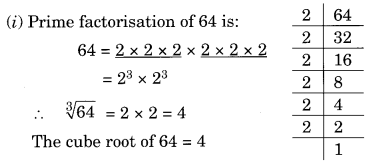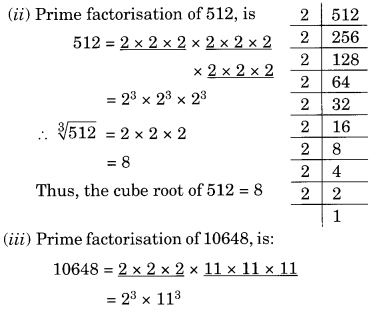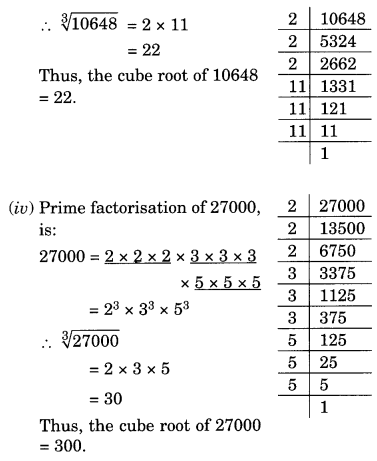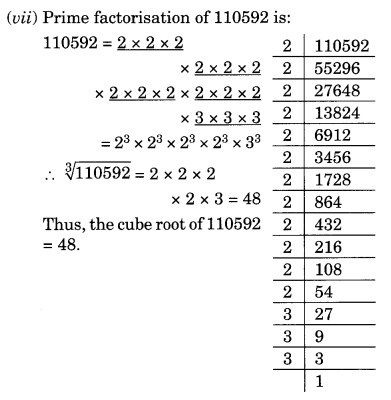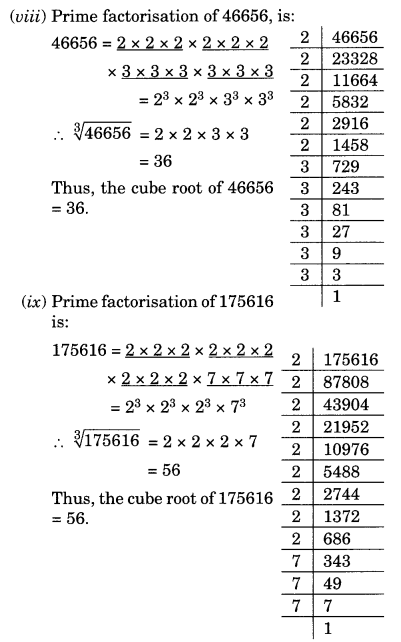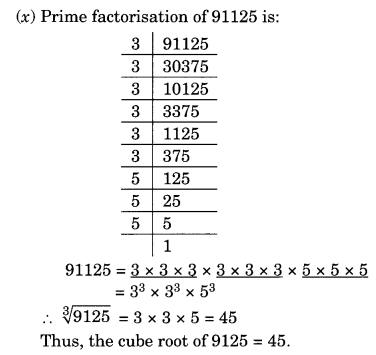Ex 7.2 Class 8 Maths Question 2.
State True or False.
(i) Cube of an odd number is even.
(ii) A perfect cube does not end with two zeros.
(iii) If the square of a number ends with 5, then its cube ends with 25.
(iv) There is no perfect cube which ends with 8.
(v) The cube of a two digit number may be a three digit number.
(vi) The cube of a two digit number may have seven or more digits.
(vii) The cube of a single digit number may be a single digit number.
Solution:
(i) False – Cube of any odd number is always odd, e.g., (7)3 = 343
(ii) True – A perfect cube does not end with two zeros.
(iii) True – If a square of a number ends with 5, then its cube ends with 25, e.g., (5)2 = 25 and (5)3 = 625
(iv) False – (12)3 = 1728 (ends with 8)
(v) False – (10)3 = 1000 (4-digit number)
(vi) False – (99)3 = 970299 (6-digit number)
(vii) True – (2)3 = 8 (1-digit number)

Ex 7.2 Class 8 Maths Question 3.
You are told that 1,331 is a perfect cube. Can you guess without factorisation what is its cube root? Similarly, guess the cube roots of 4913, 12167, 32768.
Solution:
The given perfect cube = 1331
Forming groups of three from the rightmost digits of 1331IInd group = 1
1st group = 331
One’s digit in first group = 1
One’s digit in the required cube root may be 1.
The second group has only 1.
Estimated cube root of 1331 = 11
Thus $$\sqrt [ 3 ]{ 1331 }$$ = 11
(i) Given perfect cube = 4913
Forming groups of three from the right most digit of 4913IInd group = 4
1st group = 913
One’s place digit in 913 is 3.
One’s place digit in the cube root of the given number may be 7.
Now in IInd group digit is 4
13 < 4 < 23
Ten’s place must be the smallest number 1.
Thus, the estimated cube root of 4913 = 17.
(ii) Given perfect cube = 12167
Forming group of three from the rightmost digits of 12167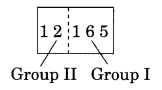We have IInd group = 12
1st group = 167
The ones place digit in 167 is 7.
One’s place digit in the cube root of the given number may be 3.
Now in Ilnd group, we have 12
23 < 12 < 33
Ten’s place of the required cube root of the given number = 2.
Thus, the estimated cube root of 12167 = 23.
(iii) Given perfect cube = 32768
Forming groups of three from the rightmost digits of 32768, we haveIInd group = 32
1st group = 768
One’s place digit in 768 is 8.
One’s place digit in the cube root of the given number may be 2.
Now in IInd group, we have 32
33 < 32 < 43
Ten’s place of the cube root of the given number = 3.
Thus, the estimated cube root of 32768 = 32.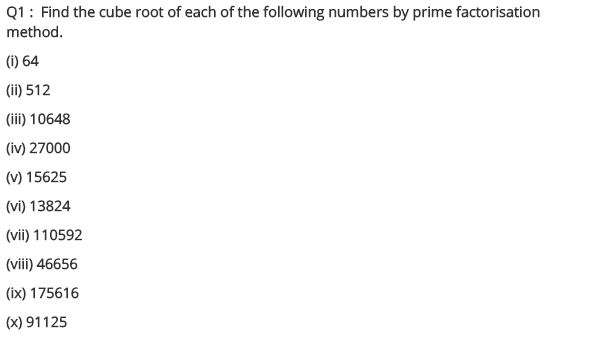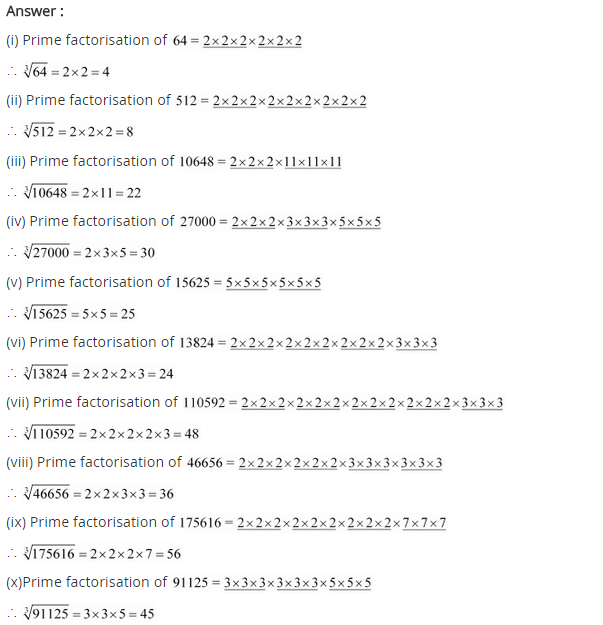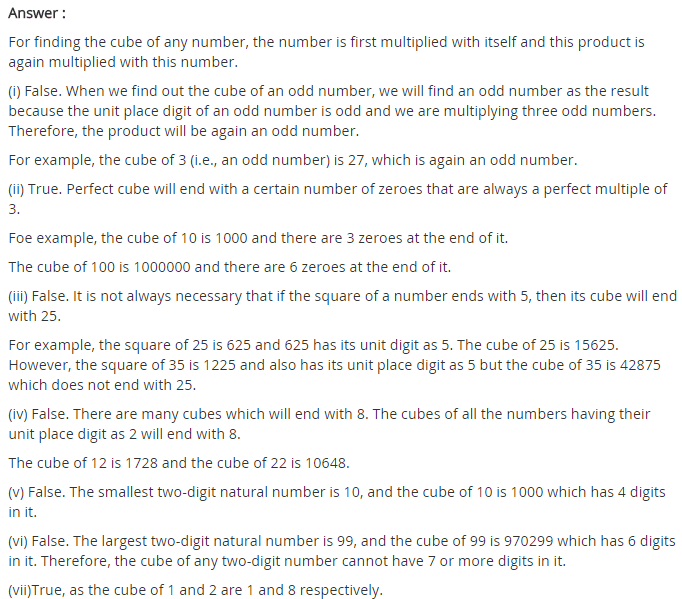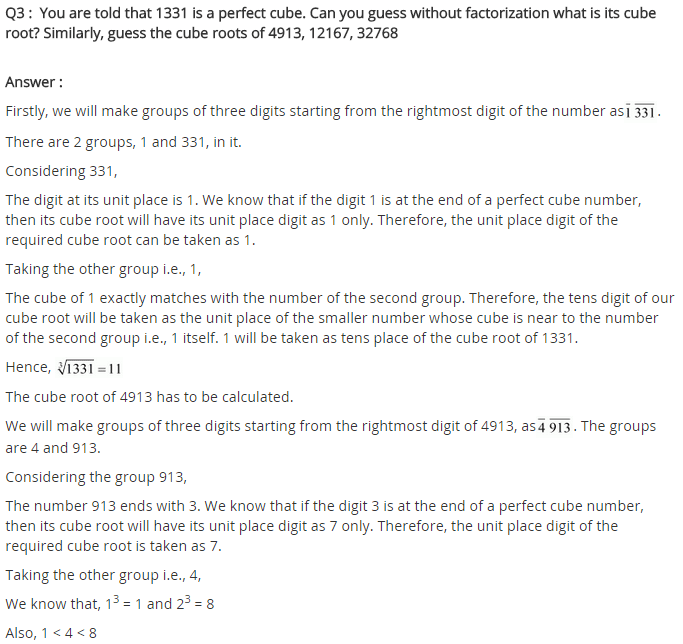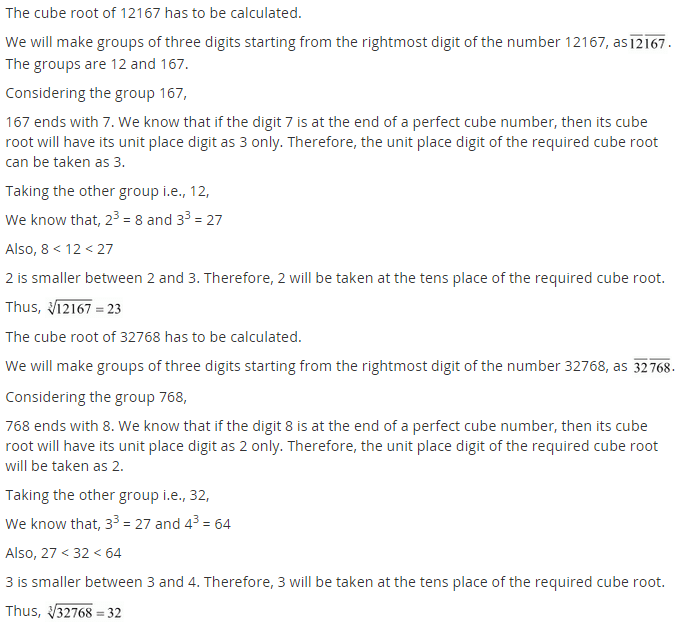+